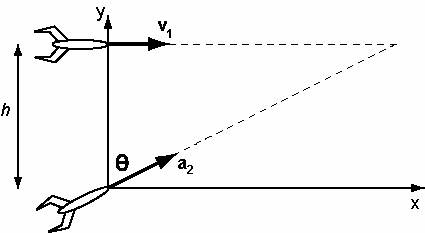# Missile Defense System - Collide Missiles

kchurchi

## Homework Statement

You are designing a missile defense system that will shoot down incoming missiles that pass over a perimeter defense post. The set-up is shown below. An incoming missile passes directly above the defense base. Radar at the base can measure the height, h, and speed, v1, of the incoming missile. Your Patriot Rocket is set to fire at an angle of θ = 46.0 degrees from vertical. You design the Patriot Rocket so the magnitude of its acceleration is given by:

a2 = A e−bt

where A can be set on your Patriot Rocket as it is fired, and b = 0.10 s-1. The direction of your Patriot Rocket's vector acceleration stays at the same angle, θ, for the entire trip. If an incoming missile passes over the defense base at a height of 4.60 km and at a constant speed of 740.0 m/s (this means that v1 is constant), solve for the value of A your Patriot Rocket must have in order to hit the incoming missile. You will also need to enter results from intermediate steps of your calculation, including the time ∆t in between launch and impact, and the horizontal distance ∆x from the launch station to the impact position.

http://lon-capa.mines.edu/res/csm/csmphyslib/Mechanics/Kinematics/2D_Projectiles/MissileDefenseSystem.jpg [Broken]

Part A) Find Δt
Part B) Find Δx
Part C) Find A

## Homework Equations

a1x(t) = 0 m/s^2
v1x(t) = v1x(0) = v1
x1(t) = v1*t

a2x(t) = a2*sin$\theta$ = A*e^(-b*t)*sin$\theta$
v2x(t) = v2x(0) -(A/b)*e^(-b*t)*sin$\theta$
x2(t) = v2x(0)*t +(A/b^2)*e^(-b*t)*sin$\theta$

a2y(t) = a2*cos$\theta$ = A*e^(-b*t)*cos$\theta$
v2y(t) = v2y(0) -(A/b)*e^(-b*t)*cos$\theta$
y2(t) = v2y(0)*t +(A/b^2)*e^(-b*t)*cos$\theta$

## The Attempt at a Solution

Part A) I know that when the missiles collide x2(t) = x1(t). I tried to use that relationship to find the change in time, but I am not sure what v2x(0) is (the initial velocity of missile 2 in the x-direction). I think as soon as I figure out part A I will be able to do the other parts. I am just a bit stuck.

Last edited by a moderator:

voko
The picture is inaccessible.

kchurchi